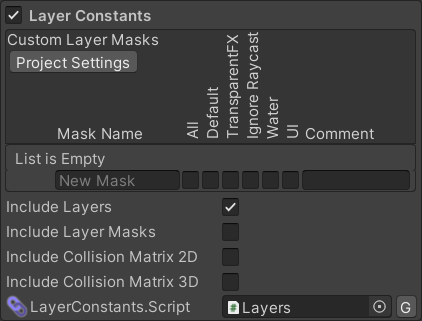﻿ Weaver - Layers

# Layers

The procedural `Layers` script contains constants corresponding to the value of each layer in your project, including any custom layers so that instead of `LayerMask.NameToLayer("MyLayer")` you can use `Weaver.Layers.MyLayer`.

# Layers PanelThe Layers panel in the Weaver Window allows the procedural script to be entirely disabled and shows the following options for customising it (Pro-Only):

Option Description
Include Layers If enabled, the script will include constants corresponding to the index of each layer.
Include Layer Masks If enabled, the script will include constants corresponding to the bit mask of each layer (`1 << LayerValue`).
Include Collision Matrix 2D If enabled, the script will include constants corresponding to the 2D collision matrix for each layer.
Include Collision Matrix 2D If enabled, the script will include constants corresponding to the 3D collision matrix for each layer.

# Sample Output

``````// This file was procedurally generated by Weaver. Any modifications will be overwritten.

#pragma warning disable // All.

namespace Weaver
{
/// <summary>This class was procedurally generated by Weaver.</summary>
public static class Layers
{
#region Layers -> Edit/Project Settings/Tags and Layers

/// <summary>Default (Mask Value = 1)</summary>
public const int Default = 0;

/// <summary>TransparentFX (Mask Value = 2)</summary>
public const int TransparentFX = 1;

/// <summary>Ignore Raycast (Mask Value = 4)</summary>
public const int IgnoreRaycast = 2;

/// <summary>Water (Mask Value = 16)</summary>
public const int Water = 4;

/// <summary>UI (Mask Value = 32)</summary>
public const int UI = 5;

/// <summary>Dynamic Obstacle (Mask Value = 256)</summary>
public const int DynamicObstacle = 8;

/// <summary>Creature (Mask Value = 512)</summary>
public const int Creature = 9;

/// <summary>Projectile (Mask Value = 1024)</summary>
public const int Projectile = 10;

/// <summary>Mini Map (Mask Value = 2048)</summary>
public const int MiniMap = 11;

#endregion

#region Custom Layer Masks -> Window/General/Weaver